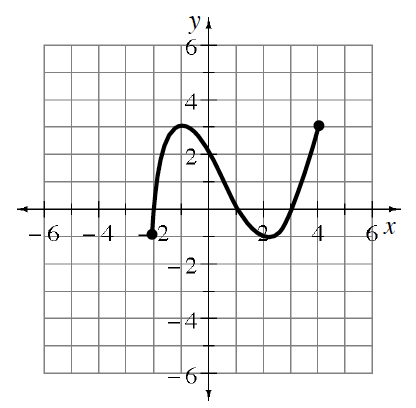### Home > CCA2 > Chapter Ch1 > Lesson 1.1.3 > Problem1-34

1-34.

Examine $g(x)$ graphed at right.

1. Which $x$-values have points on the graph? That is, describe the domain of $g(x)$.

The domain includes $−2,4$ and all the numbers between. $−2\le x\le4$.

2. What are the possible outputs for $g(x)$? That is, what is the range?

The range includes $−1,3$, and all the numbers between. $−1\le y\le3$.

3. Ricky thinks the range of $g(x)$ is: $−1,0,1,2,\ \text{and}\ 3$. Is he correct? Why or why not?

Is there a point on the graph for $x=2.5$? or for $x=−0.33$?

He is not correct since he is missing all the values between his numbers.
The curve is continuous, so the description needs to include all numbers, not just integers.

4. Draw a graph for another function with the same domain and range as $g(x)$.

There are many possibilities. Here is one example.